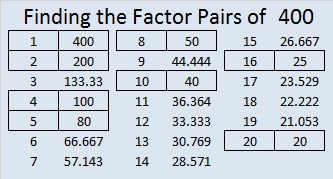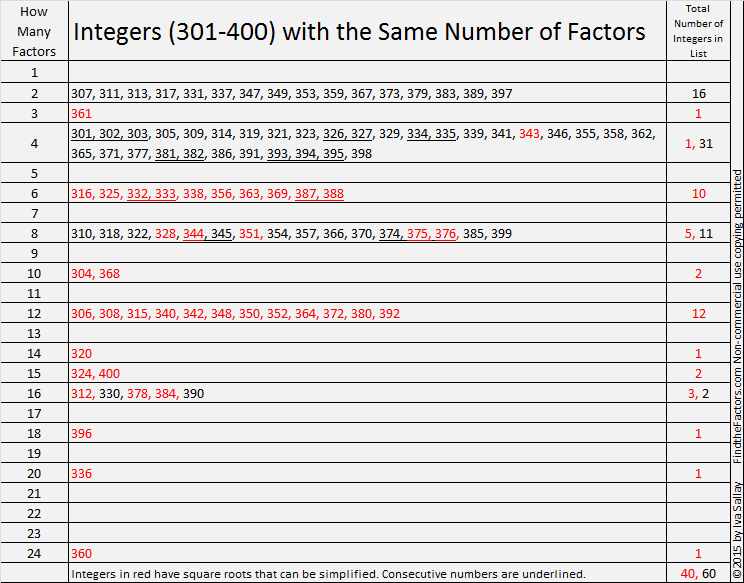# 40% of Numbers Up To 400 Have Reducible Square Roots

• 400 is a composite number.
• Prime factorization: 400 = 2 x 2 x 2 x 2 x 5 x 5, which can be written 400 = (2^4) x (5^2)
• The exponents in the prime factorization are 4 and 2. Adding one to each and multiplying we get (4 + 1)(2 + 1) = 5 x 3 = 15. Therefore 400 has exactly 15 factors.
• Factors of 400: 1, 2, 4, 5, 8, 10, 16, 20, 25, 40, 50, 80, 100, 200, 400
• Factor pairs: 400 = 1 x 400, 2 x 200, 4 x 100, 5 x 80, 8 x 50, 10 x 40, 16 x 25, or 20 x 20
• 400 is a perfect square. √400 = 20A few months ago I made a chart showing the number of factors for the first 300 counting numbers. Since this is my 400th post, I’d like to include a chart showing the number of factors for all the numbers from 301 to 400. I’m also interested in consecutive numbers with the same number of factors and whether or not the square root of a number can be reduced. The red numbers have square roots that can be reduced.The longest streak of consecutive numbers with the same number of factors is only three. There are three sets of three consecutive numbers on this chart. (Between 200 and 300 there was a streak of four consecutive numbers with six factors each.)

How do the number of factors of these 100 numbers stack up against the previous 300? The following chart shows the number of integers with a specific number of factors and how many of those integers have reducible square roots:• 39.5% or slightly less than 40% of the numbers up to 400 have reducible square roots.
• Most of these numbers have 2, 4, or 8 factors. Numbers with two factors are prime numbers. Almost all numbers with four factors are the product of two different prime numbers, and nearly two-thirds of the numbers with eight factors are the product of three different prime numbers.
• There isn’t much change between the percentages of reducible square roots from one list to the next.